Function Repository Resource:

# FullQRDecomposition

Compute the full QR decomposition of a matrix

Contributed by: Jan Mangaldan
 ResourceFunction["FullQRDecomposition"][m] yields the full QR decomposition for a numerical matrix m. The result is a list {q,r}, where q is a unitary matrix and r is an upper‐trapezoidal matrix.

## Details and Options

The original matrix m is equal to ConjugateTranspose[q].r.
q is always square, even for non-square m.
The matrix r has zeros for all entries below the leading diagonal, and has the same dimensions as m.
ResourceFunction["FullQRDecomposition"][m,PivotingTrue] yields a list {q,r,p}, where p is a permutation matrix such that m.p is equal to ConjugateTranspose[q].r.

## Examples

### Basic Examples (2)

Compute the full QR decomposition for a 3×2 matrix with exact values:

 In:=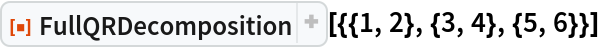Out=In:=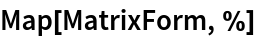Out=Compute the full QR decomposition for a 2×3 matrix with approximate numerical values:

 In:=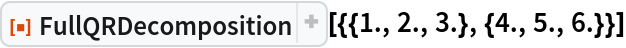Out=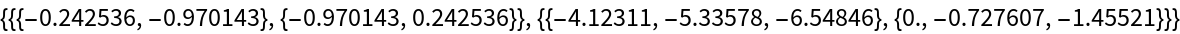In:=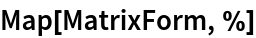Out=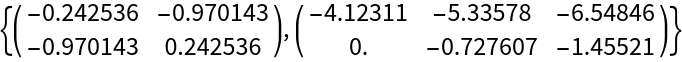### Scope (2)

Use a 3×4 matrix:Full QR decomposition computed with exact arithmetic:

 In:=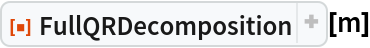Out=Full QR decomposition computed with machine arithmetic:

 In:=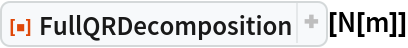Out=In:=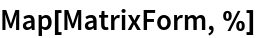Out=Full QR decomposition computed with 24-digit arithmetic:

 In:=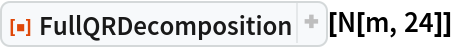Out=Full QR decomposition for a 3×3 matrix with random complex entries:

 In:=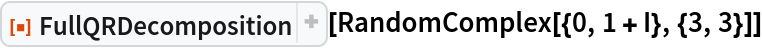Out=In:=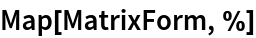Out=### Options (5)

#### Pivoting (5)

Use a 3×4 matrix:Compute the full QR decomposition using machine arithmetic with pivoting:

 In:=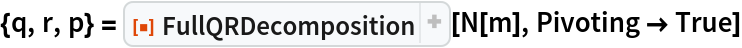Out=The elements along the diagonal of r are in order of decreasing magnitude:

 In:=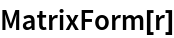Out=The matrix p is a permutation matrix:

 In:=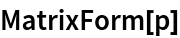Out=FullQRDecomposition satisfies m.p==ConjugateTranspose[q].r:

 In:=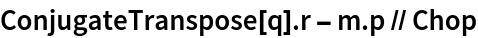Out=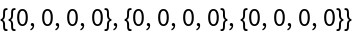### Applications (5)

Here is some data:

 In:=In:=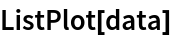Out=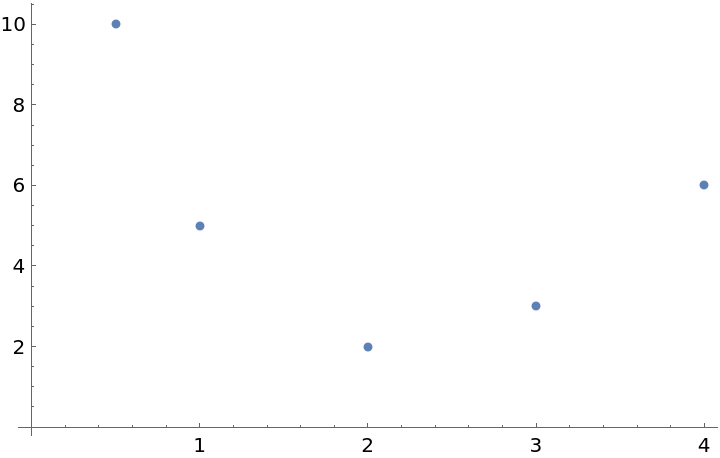Set up a design matrix for fitting with basis functions 1, t, t2:

 In:=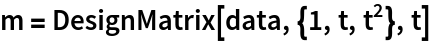Out=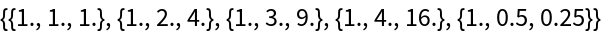Find the full QR decomposition of m:

 In:=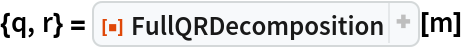Out=This finds a vector x such that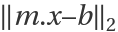is a minimum:

 In:=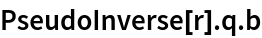Out=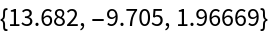These are the coefficients for the least-squares fit:

 In:=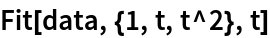Out=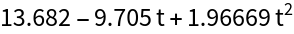In:=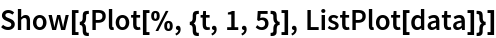Out=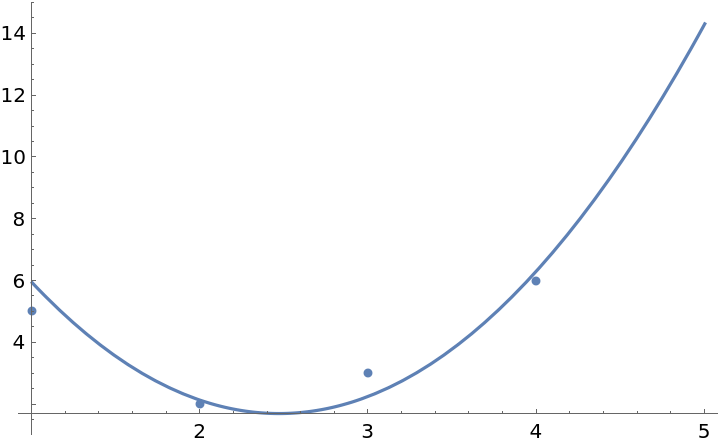### Properties and Relations (5)

Use a 3×4 matrix:

 In:=Compute the full QR decomposition:

 In:=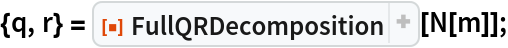The rows and columns of q are orthonormal:

 In:=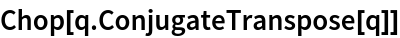Out=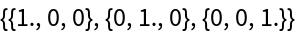In:=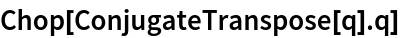Out=Check that r is upper trapezoidal:

 In:=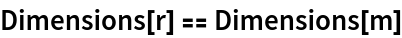Out=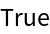In:=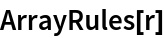Out=In:=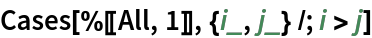Out=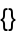Check that m is equal to ConjugateTranspose[q].r:

 In:=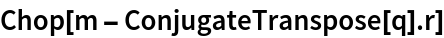Out=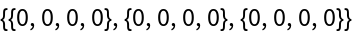## Version History

• 1.1.0 – 22 August 2022
• 1.0.2 – 16 November 2021